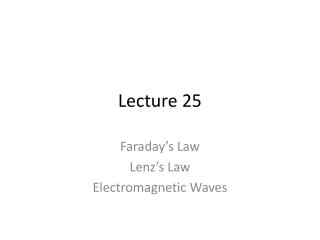DownloadDownload PresentationLecture 25

# Lecture 25

Download Presentation## Lecture 25

- - - - - - - - - - - - - - - - - - - - - - - - - - - E N D - - - - - - - - - - - - - - - - - - - - - - - - - - -
##### Presentation Transcript

1. Lecture 25 Faraday’s Law Lenz’s Law Electromagnetic Waves

2. Largest current in loop? • A • B • C • D • E • F

3. A magnetic field goes through a loop of wire, as below. If the magnitude of the magnetic field is increasing, what can we say about the current in the loop? • The loop has a clockwise current • The loop has a counter clockwise current • There is no current in the loop

4. A magnetic field goes through a loop of wire, as below. If the magnitude of the magnetic field is decreasing, what can we say about the current in the loop? • The loop has a clockwise current • The loop has a counter clockwise current • The loop has no current

5. Electromagnetic Waves Slide 25-33

6. Checking Understanding • A plane electromagnetic wave has electric and magnetic fields at all points in the plane as noted below. With the fields oriented as shown, the wave is moving • A. into the plane of the paper. • B. out of the plane of the paper. • C. to the left. • D. to the right. • E. toward the top of the paper. • F. toward the bottom of the paper. Slide 25-34

7. Electromagnetic Waves Carry Energy 1) Inside the cavity of a microwave oven, the 2.4 GHz electromagnetic waves have an intensity of 5.0 kW/m2. What is the strength of the electric field? The magnetic field? 2) A digital cell phone emits a 1.9 GHz electromagnetic wave with total power 0.60 W. At a cell phone tower 2.0 km away, what is the intensity of the wave? (Assume that the wave spreads out uniformly in all directions.) What are the electric and magnetic field strengths at this distance? Slide 25-36

8. Polarization Slide 25-37

9. Light passed through a polarizing filter has an intensity of 2.0 W/m2. How should a second polarizing filter be arranged to decrease the intensity to 1.0 W/m2? Slide 25-38

10. The Electromagnetic Spectrum Slide 25-39